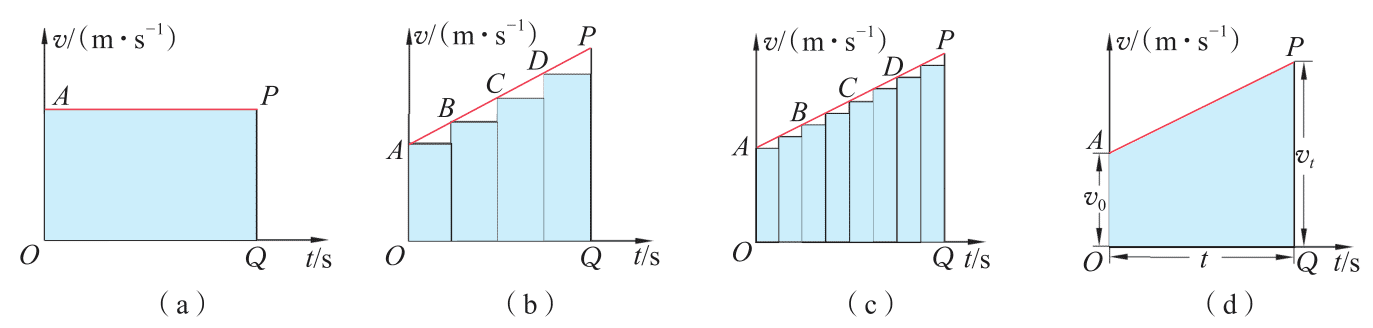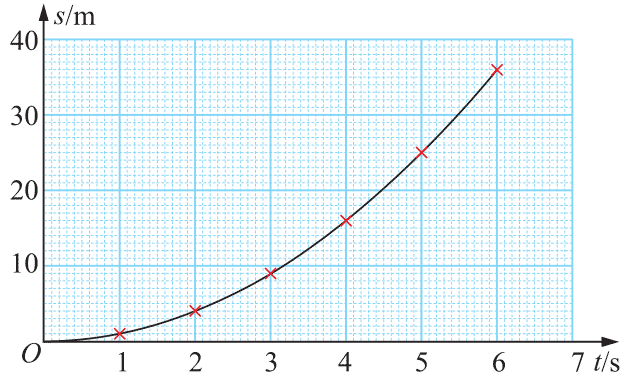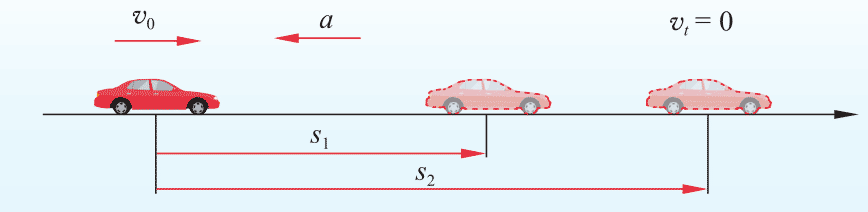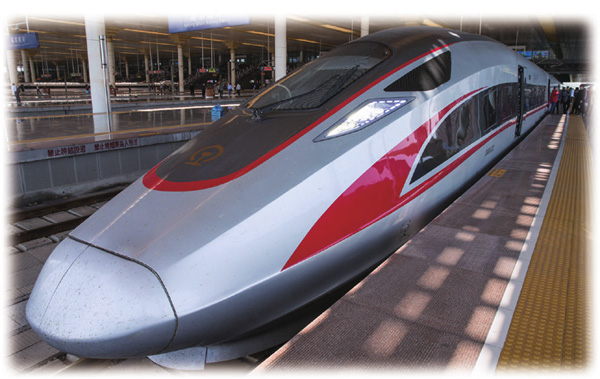# 第2章 第2节 位移变化规律

## 1．匀变速直线运动的位移-时间关系图2-7 通过v-t图像推导位移公式的示意图

$s = \frac{1}{2}({v_0} + {v_t})t$

vtv0at代入上式，有

$s = {v_0}t + \frac{1}{2}a{t^2}$

### 素养提升

——科学思维图2-8 汽车匀加速直线运动的s-t图像

s-t图像中我们可直观看出物体运动过程中位移随时间的变化情况，并可求出不同时间内物体运动的位移。

## 2．匀变速直线运动的位移-速度关系

$v_t^2 - v_0^2 = 2as$

### 例题图2-9 汽车匀减速直线运动过程示意图

${t_0} = \frac{{{v_t} - {v_0}}}{a} = \frac{{0 - 27.8{\rm{m/s}}}}{{ - 5{\rm{m/}}{{\rm{s}}^{\rm{2}}}}} = 5.56{\rm{s}}$

$\begin{array}{l}{s_1} = {v_0}t + \frac{1}{2}a{t^2}\\ = 27.8{\rm{m/s}} \times 2{\rm{s}} + \frac{1}{2} \times ( - 5{\rm{m/}}{{\rm{s}}^{\rm{2}}}) \times {(2{\rm{s}})^2}\\ = 45.6{\rm{m}}\end{array}$

$\begin{array}{l}{s_2} = \frac{{v_t^2 - v_0^2}}{{2a}}\\ = \frac{{0 - {{(27.8{\rm{m/s}})}^2}}}{{2 \times ( - 5{\rm{m/}}{{\rm{s}}^{\rm{2}}})}}\\ = 77.3{\rm{m}}\end{array}$

——物理观念

## 节练习

1．一辆电动车以4 m/s的速度沿直线匀速行驶，然后以1 ms2的加速度匀加速行驶。求电动车匀加速行驶10 m时的速度。

【解答】6 m/s

2．若某汽车以18 m/s的速度行驶，刹车后做匀减速直线运动，加速度大小为4 m/s2，求刹车后5 s内汽车前进的距离。

【解答】40.5 m

3．如图所示，在某一段平直的铁路上，一列以144 km/h的速度行驶的动车开始匀减速行驶，经过2 min，动车恰好停在车站；在车站停留4 min，动车匀加速驶离车站，离开车站2 km时恢复到原来的速度144 km/h，求：第3题

（1）动车减速时的加速度大小；

（2）动车从开始减速到恢复到原速度这段时间内的平均速度大小。

【解答】（1）a＝－0.33 m/s2

（2）9.6 m/s

4．某型号的汽车在以72 km/h的速率行驶时，可以在54 m的距离内停下来；在以36 km/h的速率行驶时，可以在17 m的距离内停下来。假设两次刹车中，驾驶员的反应时间和刹车的加速度都相同，求驾驶员的反应时间和刹车时加速度的大小。

【解答】t＝0.7 s，a＝5 m/s2

5．试证明：做匀变速直线运动的物体在一段时间内的平均速度等于这段时间中间时刻的瞬时速度。

*6．做匀变速直线运动的物体，设在各个连续相等的时间t内的位移分别是s1s2s3，…，sn。如果物体的加速度是a，试证明：

（1）s2s1s3s2＝…＝snsn－1at2

（2）s4s1s5s2s6s3＝3at2